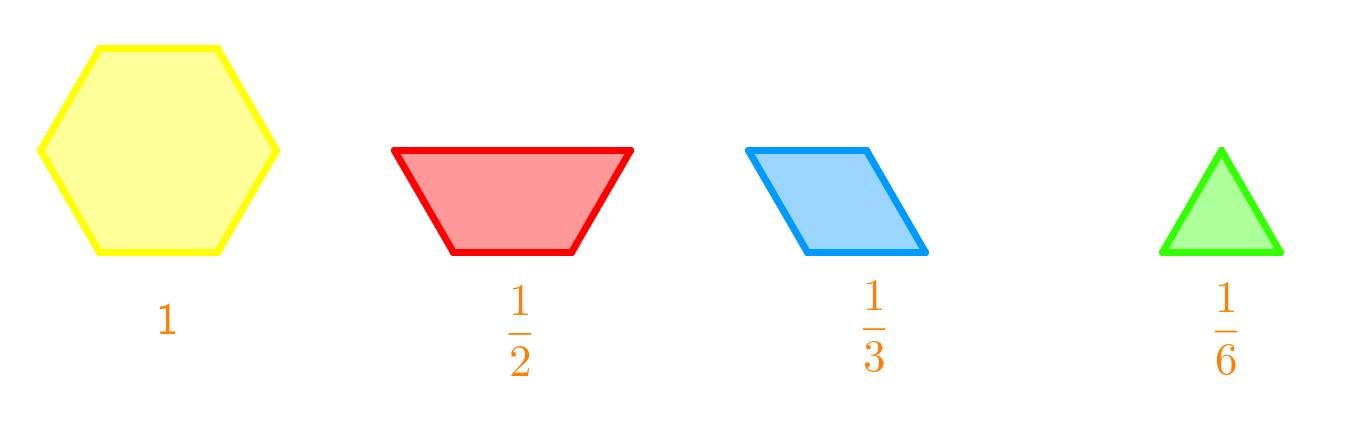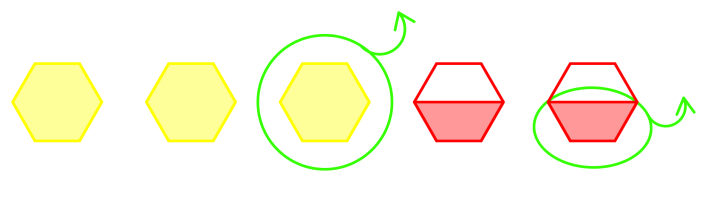# Adding and subtracting mixed numbers

### Adding and subtracting mixed numbers

In this section, we will write addition and subtraction statements for mixed numbers using given diagrams. First, we will add and subtract the whole numbers. Second, we will add and subtract the fractions. Some of the fractions will have like denominators and others will have unlike denominators. Before adding and subtracting those fractions with unlike denominators, we will have to determine the lowest common denominator for the pair of fractions and then write the equivalent fractions. We will write any resulting improper fractions in our answers as mixed numbers.

#### Lessons

• Introduction
a)
Simplify fractions: Method A - By using greatest common factors

b)
Simplify fractions: Method B - By using common factors

c)
What are equivalent fractions?

d)

e)
How to subtract frations?
• subtracting with improper fractions
• subtracting with mixed numbers

f)
Proper fractions VS. Improper fractions VS. Mixed fractions

• 1.
Subtract or add using the given diagram.a)b)• 2.
$1\frac{3}{6}+\frac{3}{8}$
Maren goes to soccer practice for $2\frac{4}{5}$ hour each day. In the morning, she has $1\frac{3}{4}$ hour of practice. How many hours of practice does she have in the afternoon?
Hannah ran for $1\frac{1}{4}$ hour and then biked for $1\frac{2}{3}$ hour. For how long did she exercise?